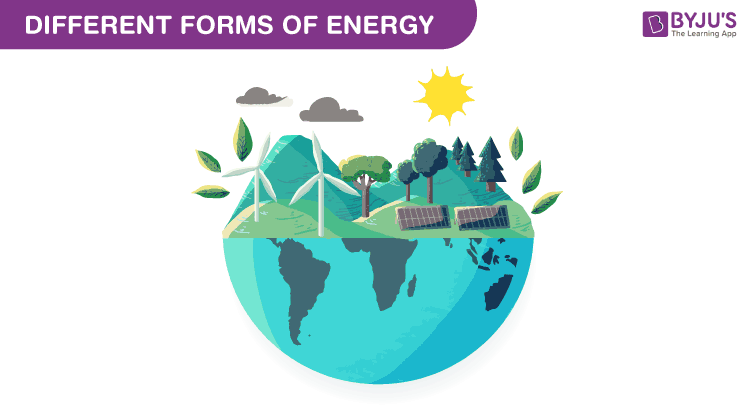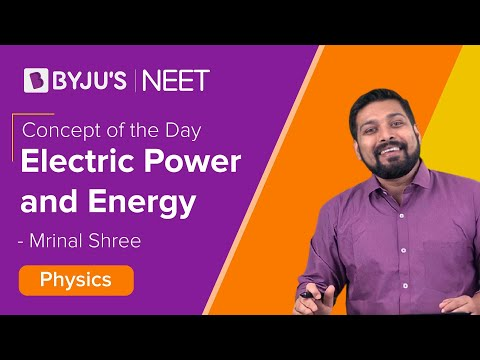# Different Forms Of Energy

## What is Energy?

We can define energy as the capacity to do work. There are various forms of energy. The law of conservation of energy states that energy can neither be created nor be destroyed. It can only be transferred from one form to another. Some forms of energy are defined and discussed in this article.### Heat Energy

When you leave a cup of hot coffee unattended for some time, you find that the coffee has cooled down. This is because it loses heat to the surrounding which is at a lower temperature when compared to the coffee.

Heat energy is the form of energy that results from the total kinetic energy of the particles in any object. It is transferred as a result of a random motion of molecules which leads to them bouncing off each other and transferring energy in the process. It is also sometimes referred to as the energy in transit. Heat flows from a body of higher temperature (high total kinetic energy of the particles) to a body of lower temperature (low total kinetic energy of the particles).

### Chemical Energy

Plants use solar energy, carbon dioxide, and water to synthesize food. This food is consumed by humans and animals, is broken down in their bodies, and they get energy for life activities. They are all examples of chemical energy.

Chemical energy is the energy that is stored in the bonds of chemical compounds. Moreover, a chemically stable compound has less energy stored in it as compared to its separate parts. When a chemical reaction takes place, there is a change in the arrangement of atoms which results in either absorption (endothermic) or a release (exothermic) of energy.

### Electrical Energy

The energy due to the moving electrical charges is called electrical energy. So when you turn on a torch, the chemical energy stored in batteries makes the charged particles flow from the low potential terminal to a high potential terminal. This electrical energy lights the torch, which is transferred to the surrounding as light energy.

### Nuclear Energy

You might be aware of the infamous bombings of the cities of Hiroshima and Nagasaki during World War II which caused the destruction to the extent that its consequences can be seen even today. Those were the nuclear bombs which are considered as one of the most dangerous inventions of mankind till date.
Nuclear energy is the energy in the nucleus of an atom. The energy is released during nuclear fusion or fission reactions. And the energy released is really vast. When the reaction is controlled, it can be used to generate electricity. Under uncontrolled nuclear fission reactions, nuclear weapons are created.

### The Equivalence of Mass and Energy

An equation given by Einstein explains the relationship equivalence of mass and energy. According to it, the energy of a body is equal to the products of the mass of the body and the square of the speed of light. The famous equation is

E = mc2

Where E is the energy, m is the mass of the body and c is the speed of light in a vacuum which is approximately equal to 3 x 108 m/sec.

Stay tuned with BYJU’S to learn more about energy, nuclear fusion, energy conversion, and more with interesting video lectures.

## What is Electrical Power?## Frequently Asked Questions – FAQs

Q1

### Define energy.

We can define energy as the capacity to do work. There are various forms of energy.
Q2

### What is the law of conservation of energy?

The law of conservation of energy states that energy can neither be created nor be destroyed. It can only be transferred from one form to another.
Q3

### What is chemical energy?

Chemical energy is the energy that is stored in the bonds of chemical compounds. Moreover, a chemically stable compound has less energy stored in it as compared to its separate parts.
Q4

### What is mass and energy equivalence?

An equation given by Einstein explains the relationship equivalence of mass and energy. According to it, the energy of a body is equal to the products of the mass of the body and the square of the speed of light. The famous equation is
E = mc2
Where E is the energy, m is the mass of the body and c is the speed of light in a vacuum which is approximately equal to 3 x 108 m/sec.
Q5

### What is nuclear energy?

Nuclear energy is the energy in the nucleus of an atom. The energy is released during nuclear fusion or fission reactions. And the energy released is really vast. When the reaction is controlled, it can be used to generate electricity.
Test Your Knowledge On Forms Of Energy!